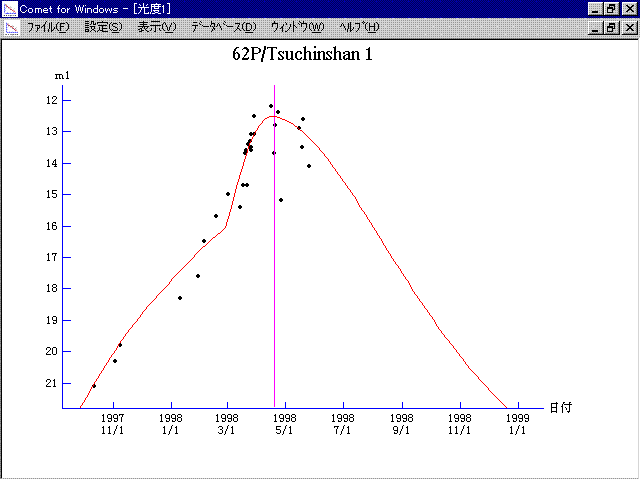# 62P/Tsuchinshan 1 (1998)###Orbital Elements

```   The following improved orbital elements by Kenji Muraoka,
are from 130 observations 1965 to 1998, including 9 Planets,
Moon and 5 minor planets perturbations and non-gravitational
effect of style II.  The mean residual is +/- 1.00 arc seconds.

Epoch  =  1998 Apr. 17.0  TT       JDT = 2450920.5
T  =  1998 Apr. 19.05196       +/- 0.00047 (m.e.) TT
Peri. =   22.76976                +/- 0.00033
Node  =   96.81028                +/- 0.00011   (2000.0)
Incl. =   10.49530                +/- 0.00002
q  =    1.4958547              +/- 0.0000011 AU
e  =    0.5766105              +/- 0.0000004
a  =    3.5330461              +/- 0.0000012 AU
n  =    0.14841580             +/- 0.00000007
P  =    6.641                  +/- 0.0000033  years
A1  =   +0.302                  +/- 0.016
A2  =   +0.00912                +/- 0.00005
```

###Finding Charts1997 Sept. 9 - 1998 June 161998 May 7 - 1998 Nov. 3

###Magnitudes Graph

```        m1 =   9.0 + 5 log d +  30.0 log r  [   ,-50]  (             - 1998 Feb. 28)
m1 = -15   + 5 log d + 150   log r  [-50,  0]  (1998 Feb. 28 - 1998 Apr. 19)
m1 =   6.0 + 5 log d +  30.0 log r  [  0,   ]  (1998 Apr. 19 -             )
```##### The orbital elements are calculated by Kenji Muraoka. The charts are made with StellaNavigator Ver.2.0 for Windows (AstroArts / ASCII). The magnitudes graphs are made with Comet for Windows.